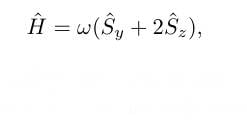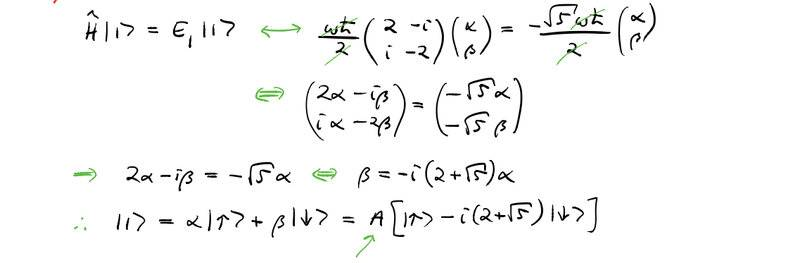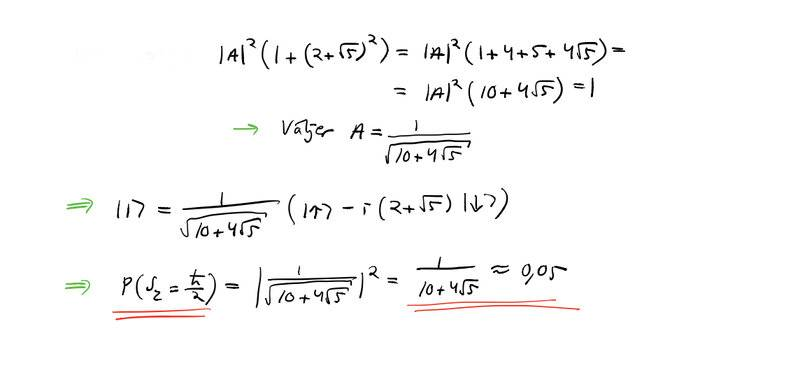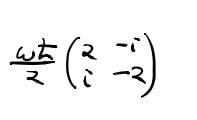# Intro to quantum mechanics - Spin and linear algebra

• Graham87
The matrix is the representation of the operator ##\hat{H}## in the eigenbasis of ##\hat{s}_z##. You must distinguish between operators, living in the abstract 2D Hilbert space and their components wrt. some basis. Then all this confusion wouldn't occur.f

#### Graham87

Homework Statement
Measure Sz from the following expression see pic.
Relevant Equations
spin=1/2
Operators Sy and Sz represent standard spin vectors and w is a positive constant.So this expression is apparently in Sz basis? How can you see that?
How would it look in Sy basis for example?
The solution is following. They are putting Sz as a basis, bur how do you know that Sz is the basis here?

ThanksYou know it's the z basis because of the matrix form of the operators.

•topsquark and Graham87
You know it's the z basis because of the matrix form of the operators.
Aha, so what signifies z basis? Is it this:It looks like z and y basis added together?
How would a y basis look?

Thanks!
I will polish my linear algebra soon.

The matrix representing ##S_z## is a diagonal matrix with ##\pm \frac \hbar 2## on the diagonals. That indicates the z basis.

•topsquark and Graham87
The matrix representing ##S_z## is a diagonal matrix with ##\pm \frac \hbar 2## on the diagonals. That indicates the z basis.
Yes. They are asking for Sz of H, so I thought you first need to make a basis change with Sz basis. I think they did that in the solution. But I can’t tell what basis it is. I thought alpha and beta are the new basis matrix?

The first thing they did was express ##H## in the z basis, using the familiar form of ##S_y## and ##S_z## in that basis.

Next, they find the eigenvalues and eigenvectors of ##H##, with the vectors represented in the z basis.

•topsquark and Graham87
The first thing they did was express ##H## in the z basis, using the familiar form of ##S_y## and ##S_z## in that basis.

Next, they find the eigenvalues and eigenvectors of ##H##, with the vectors represented in the z basis.
Aha, I don’t get howis expressed in the z basis. It looks like the diagonal of z and diagonal of y.

Aha, I don’t get how View attachment 313217is expressed in the z basis. It looks like the diagonal of z and diagonal of y.
I suspect you don't understand what it means for a matrix to be expressed in a particular basis.

You seem to be leaning towards wanting to express ##H## in its own eigenbasis.

•Graham87 and topsquark
The matrix is the representation of the operator ##\hat{H}## in the eigenbasis of ##\hat{s}_z##. You must distinguish between operators, living in the abstract 2D Hilbert space and their components wrt. some basis. Then all this confusion wouldn't occur. The matrix elements are by definition ##H_{\sigma_1 \sigma_2} = \langle \sigma_1 | \hat{H} | \sigma_2 \rangle##.

•Graham87 and malawi_glenn